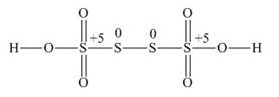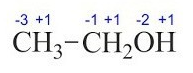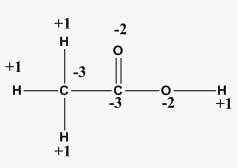# 8.2 What are the oxidation number of the underlined elements in each of the following and how do you rationalize your results

Solution-

O.N of potassium ()= +1

O.N of hydrogen()= +1 (In case of metalic hydride, -1)

O.N of Oxygen() = -2 ( In case of peroxide and superoxide it wil be different ON)

1*1 + 3*x = 0

x = (-1/3)

average  O. N. Of is    . But it is wrong because  O.N cannot be fractional. So lets try with structure of

O.N of  = -1 (because a coordinate bond is formed between   and    ion. Hence O. N of three atoms are 0,0 and -1, O.N 0 in moleculeand -1 in  ion.)

Assume O.N of S is x

Fractional O.N  is not possible so try with structure -The two S atom present in the middle has zero (0) O.N and other two have (+5) O.N [ -2 from two O atom and -1 from OH]

If you calculate the oxidation number of Fe in  it would be 8/3 and however, O.N cannot be in fractional.

Here one iron atom has +2 O.N and the other two are of +3 O.N.

let assume carbon has x oxidation Number

So,[ x + 1(3) +x +1(2) +(-2)+1 = 0]

2x = -4

x=-2

In this molecule two carbon atoms present in different enviroments. Hence, they cannot have the same O.N.Thus, C exhibits the O. S  of -3 and -1.suppose the oxidation number of Carbon is x.

If we calculate the O.N of x we get x=0

However, 0 is average O.N. of C atoms. In this molecule two carbon atoms present in different enviroments. Hence, they cannot have the same O.N.Thus, C exhibits the O.S  of +3 and 3 in CH3COOH This can be more understood by structure-Here we can see that at right C, +3 O.N (-1 from OH and -2from O atom) and in left C, -3 O.N(contribution from H atom only)

## Related Chapters

### Preparation Products

##### JEE Main Rank Booster 2021

This course will help student to be better prepared and study in the right direction for JEE Main..

₹ 13999/- ₹ 9999/-
##### Rank Booster NEET 2021

This course will help student to be better prepared and study in the right direction for NEET..

₹ 13999/- ₹ 9999/-
##### Knockout JEE Main April 2021 (Subscription)

An exhaustive E-learning program for the complete preparation of JEE Main..

₹ 4999/-
##### Knockout NEET May 2021

An exhaustive E-learning program for the complete preparation of NEET..

₹ 22999/- ₹ 14999/-